# Kerala Syllabus 9th Standard Maths Solutions Chapter 7 Similar Triangles

## Kerala State Syllabus 9th Standard Maths Solutions Chapter 7 Similar Triangles

### Kerala Syllabus 9th Standard Maths Similar Triangles Text Book Questions and Answers

Textbook Page No. 100

Similar Triangles Class 9 Kerala Syllabus Question 1.
The perpendicular from the square corner of a right triangle cuts the opposite side into two parts of 2 and 3 centimetres length.i. Prove that the two small right triangles cut by the perpendicular have the same angles.
ii. Taking the length of the perpendicular as h, prove that $$\frac{h}{2} = \frac{3}{h}$$
iii. Calculate the perpendicular sides of the large triangle.
iv. Prove that if the perpendicular from the square corner of a right triangle divides the opposite side into parts of lengths a and b and if the length of the perpendicular is h, then h² = ab.
∠DAC = ( 90 – x)°∴ ∠B = 180 – (x + 90) = 90 – x
and ∠C = 180 – (90 – x + 90) = x
Angles of ΔABC = x°, (90 – x)°, 90°
Angles of ΔACD = x°, (90 – x)°, 90°
The two small triangles have the same angles.

ii. Triangle with the same angles, sides opposite equal angles are scaled by the factor.
2 : h = h : 3 ⇒ $$\frac{2}{h} = \frac{h}{3}$$
i.e $$\frac{h}{2} = \frac{3}{h}$$

iii. $$\frac{h}{2} = \frac{3}{h}$$
h² = 6 ⇒, h = $$\sqrt 6$$
In ΔABD
AB² = BD² + AD² = 2² + ($$\sqrt 6$$)²
= 4 + 6 = 10
AB = $$\sqrt 10$$
AC² = DC² + AD² = 3² + ($$\sqrt 6$$)²
= 9 + 6 = 15
AC = $$\sqrt 15$$
The perpendicular sides are $$\sqrt 10$$ and $$\sqrt 15$$

iv. Triangle with the same angles, sides opposite equal angles are scaled by the same factor.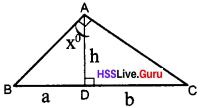$$\frac{h}{a} = \frac{b}{h}$$
h² = ab

Similar Triangles Class 9 Question 2.
At two ends of a horizontal line, angles of equal size are drawn and two points on the slanted lines are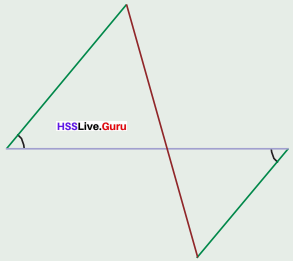i. Prove that the parts of the horizontal line and parts of the slanted line are in the same ratio.
ii. Prove that the two slanted lines at the ends of the horizontal line are also in the same ratio.
iii. Explain how a line of length 6 cm can be divided in the ratio 3 : 4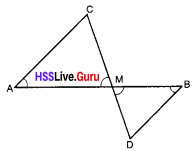i. ∠A = ∠B
∠AMC = ∠BMD
∴ ∠C = ∠D
(The triangles AMC and DMB are similar).
∴ $$\frac{MC}{MD} = \frac{AC}{BD} = \frac{AM}{MB}$$
$$\frac{AM}{MB} = \frac{MC}{MD}$$

ii. $$\frac{MC}{MD} = \frac{AC}{BD} = \frac{AM}{MB}$$
(From the similar triangles AMC and DMB)

iii. Draw a line segment of length 6 cm. At one end of this line AB, 3cm long and at the other end draw CD, 4cm long in the opposite direction.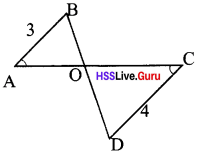∠CAB = ∠ACD
Draw BD to cut A at O.
Since AB : CD = OA : OC
∴ OA : OC = 3 : 4

Scert Class 9 Maths Similar Triangles  Question 3.
The mid point of the bottom side of a square is joined to the ends of the top side and extended by the same length. The ends of these lines are joined and perpendiculars are drawn from these points to the bottom side of the square extended.i. Prove that the quadrilateral obtained thus is also a square.
ii. Explain how we can draw a square with two corners on a semicircle and the other two corners on its diameter as in the figure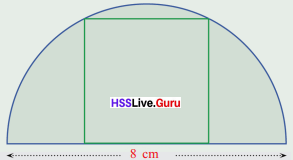i. ΔDCM and ΔSRM are similar.
The angles of the ΔMBC and ΔMAD are equal.
ΔPMS, ΔQMR are also similar triangles.
∠D = ∠S; ∠C = ∠R; ∠A = ∠B; ∠B = ∠Q
Let the ratio of the equal sides are be k.
$$\frac{QR}{BC}$$ = k ⇒ $$\frac{QR}{2a}$$ = k
∴ QR = 2ak
Similarly, PS = PQ = SR = 2ka
∴ PQRS is a square.

ii.Draw a line of AB, 8cm long. Find midpoint O on the line. Mark P and Q such that OP = OQ = 1cm. Draw the square PQRS whose sides are 2cm long.

Draw a semicircle with O as centre and OA as radius. Extend OS and OR to meet the semicircle at G and F. From G and F draw GD and FE perpendicular AB. Draw GF. Quadrilateral DEFG is the required square.

Kerala Syllabus 9th Standard Maths Chapter 7 Question 4.
The picture shows a square drawn sharing one corner with a right triangle and the other three corners on the sides of this triangle.i. Calculate the length of a side of the square.
ii. What is the length of a side of the square drawn like this within a triangle of sides 3, 4 and 5 centmetres?
i.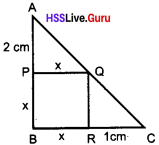ΔABC and ΔAPQ are similar.
$$\frac{x + 2}{2} = \frac{x + 1}{x}$$ = x(x + 2) = 2(x +1)
x² + 2x = 2x + 2
x² = 2; x = $$\sqrt 2$$
Side of square = $$\sqrt 2$$cm.

ii. AB = 4, BC = 3, AC = 5
ΔAPQ and ΔABC are similar triangles
∴ $$\frac{AP}{PB} = \frac{PQ}{BC} ⇒ \frac{4 – x}{4} = \frac{x}{3}$$
3(4 – x) = 4x
12 – 3 = 4x
12 – 7x; x = $$\frac {12}{7}$$The length of the side of the square = $$1\frac {5}{7}$$

Chapter 7 Similar Triangles Kerala Syllabus Question 5.
Two poles of heights 3 m and 2 m are erected upright on the ground and ropes are stretched from the top of each to the foot of the other.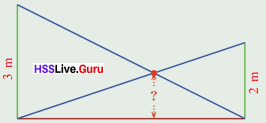i. At what height above the ground do the ropes cross each other?
ii. Taking the heights of the poles as a and b and height above the ground of the point where the ropes cross each other as h, And the relation between a, b and h.
iii. Prove that this height would be the same, whatever be the distance between the poles.
i. Consider ΔABC and ΔAFE. They are similar.
$$\frac{b}{h} = \frac{x + y}{x}$$ ………..(1)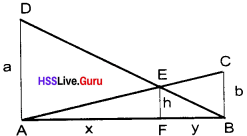Consider ΔADB and ΔFEB. They are also similar
$$\frac{a}{h} = \frac{x + y}{y}$$ ………..(1)
$$\frac{h}{a} = \frac{y}{x + y}, \frac{h}{b} = \frac{x}{x + y}$$
(1) + (2) ⇒ $$\frac{h}{a} + \frac{h}{b} = \frac{y}{x + y} + \frac{x}{x + y}$$
h$$(\frac{1}{a} + \frac{1}{b}) = \frac{x + y}{x + y}$$
h$$(\frac{1}{a} + \frac{1}{b})$$ = 1
$$\frac{1}{a} + \frac{1}{b} = \frac{1}{h}$$ ……..(2)
a = 3 and b = 2
$$\frac{1}{h} = \frac{1}{3} + \frac{1}{2} + \frac{5}{6}$$cm
h = $$\frac{6}{5}$$ = 1.2

ii. The heights of the poles are a and b and the height above the ground of the point where the ropes cross each other is h, then the relation between a, b and h is $$\frac{h}{a} + \frac{h}{b}$$ = 1 or $$\frac{a + b}{ab} = \frac{1}{h}$$ from equation (3).

iii. Only change its height according to the height of the poles not the distance.

Textbook Page No. 107

9th Class Maths Notes Kerala Syllabus Question 1.
Draw a triangle of angles the same as those of the triangle shown and sides scaled by $$1\frac{1}{4}$$One side is 6 cm. Its $$1\frac{1}{4}$$ part is 7.5 cm
Other sides are 4 × $$1\frac{1}{4}$$ = 5 cm
8 × $$1\frac{1}{4}$$ = 10 cm
Draw one side in 10 cm. Complete the triangle with given measures.Kerala Syllabus Class 9 Maths Solutions Question 2.
See this picture of a quadrilateral.i. Draw a quadrilateral with angles the same as those of this one and sides scaled by $$1\frac{1}{2}$$
ii. Draw a quadrilateral with angles different from those of this and sides scaled by $$1\frac{1}{2}$$.
i. Draw a quadrilateral by increasing all the sides including the diagonal by $$1\frac{1}{2}$$
4 × $$1\frac{1}{2}$$ = 4 × 1.5 = 6
2 × $$1\frac{1}{2}$$ = 2 × 1.5 = 3
3 × $$1\frac{1}{2}$$ = 3 × 1.5 = 4.5
5 × $$1\frac{1}{2}$$ = 5 × 1.5 = 7.5
6 × $$1\frac{1}{2}$$ = 6 × 1.5 = 9ii. Draw a quadrilateral by increasing all the sides increased by $$1\frac{1}{2}$$ except the diagonal.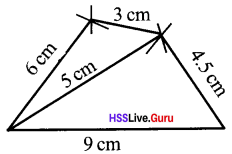Textbook Page No. 111

Kerala Syllabus 9th Standard Maths Notes Question 1.
The picture shows two circles with the same centre and two triangles formed by joining the centre to the points of intersection of the circles with two radii of the larger circle. Prove that these triangles are similar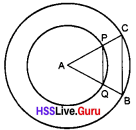∠APQ = ∠AQP
(Base angles of an isosceles triangle)
∠A = ∠A (Common angle)
∴ ∠ACB = ∠ABC
∴ $$\frac{AP}{AC} = \frac{AQ}{AB}$$
That is two sides of these triangles are scaled by the same factor.
Since two sides of these triangles are scaled by the same factor and the angle between them the same ΔAPQ and ΔACB are similar. So the triangles are similar

Kerala Syllabus 9th Standard Maths Guide In Malayalam Question 2.
The lines joining the circumcentre of a triangle to the vertices are extended to meet another circle with the same centre and these points are joined to make another triangle.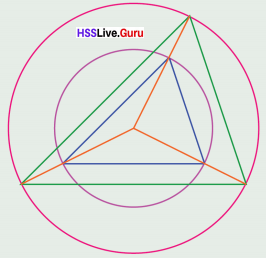i. Prove that the two triangles are similar.
ii. Prove that the scale factor of the sides of the triangle is the scale factor of the radii of the circles.
∴ ΔAOB and ΔPOQ are similar triangles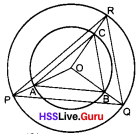∴ $$\frac{AB}{PQ} = \frac{OB}{OQ}$$ ……..(1)
ΔBOC, ∠QOR are similar
∴ $$\frac{OB}{OQ} = \frac{BC}{QR} = \frac{OC}{OR}$$ ……..(2)
Similarly
$$\frac{OC}{OR} = \frac{AC}{PR}$$ ……..(3)
(1), (2), (3) consider
∴ $$\frac{AB}{PQ} = \frac{BC}{QR} = \frac{AC}{PR}$$
ΔABC and ΔPQR are similar triangles.

ii. From equation (1), (2) and (3)
The scale factor of the sides of the triangle is the scale factor of the radii of the circles.

9th Class Maths Kerala Syllabus Question 3.
A point inside a quadrilateral is joined to its vertices and the lines are extended by the same scale factor. Their ends are joined to make another quadrilateral.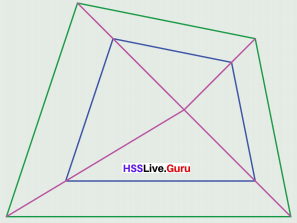i. Prove that the sides of the two quadrilateral are scaled by the same factor.
ii. Prove that the angles of the two quadrilaterals are the same.
i.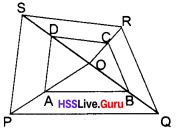ΔAOB, ΔPQO are similar
$$\frac{OA}{OP} = \frac{AB}{PQ} = \frac{OB}{OQ}$$ …….(1)
ΔOBC, ΔOQR are similar
$$\frac{OB}{OQ} = \frac{BC}{QR} = \frac{OC}{OR}$$ …….(2)
ΔOCD, ΔORS are similar
$$\frac{OC}{OR} = \frac{CD}{RS} = \frac{OD}{OS}$$ …….(3)
ΔODA, ΔOSP are similar
$$\frac{OD}{OS} = \frac{AD}{PS} = \frac{OA}{OP}$$ …….(4)
Consider (1), (2), (3), (4)
$$\frac{AB}{PQ} = \frac{BC}{QR} = \frac{DC}{SR} = \frac{AD}{PS}$$
Since the sides of quadrilateral EFGH and quadrilateral ABCD are scaled by the same factor.
∴ The angles of the quadrilateral are also same (Angles of similar triangles).

ii. Since the sides of quadrilateral PQRS and quadrilateral ABCD are scaled by the same factor, the angles of the large quadrilateral will be the same as the angles of the small quadrilateral.

### Kerala Syllabus 9th Standard Maths Similar Triangles Exam Oriented Text Book Questions and Answers

Class 9 Maths Notes Kerala Syllabus Question 1.ΔABC, ΔXYZ are similar triangles in the picture. Then
$$\frac{AB}{XY} = \frac{BC}{….} = \frac{….}{XZ}$$
$$\frac{AB}{XY} = \frac{BC}{YZ} = \frac{AC}{XZ}$$

Hss Live Class 9 Maths Kerala Syllabus Question 2.
In ΔXYZ PQ is parallel to XY.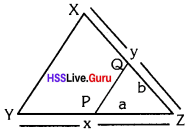a. $$\frac{YP}{PZ} = \frac{XQ}{….}$$
b. $$\frac{x}{a} = \frac{…..}{b}$$
a. $$\frac{YP}{PZ} = \frac{XQ}{QZ}$$
b. $$\frac{x}{a} = \frac{y}{b}$$

Kerala Syllabus 9th Standard Maths Solutions Question 3.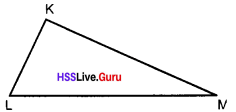Fill in the blanks according to the picture.
∠K = ∠A; ∠B = …….
∠C = ……; KL = ……cm
KM = ….. cm
∠B = ∠L
∠C = ∠M
KL = 8cm
KM = 10cm

9th Standard Maths Notes Kerala Syllabus Question 4.
In the diagram AP and BQ are perpendicular to AB. AP = 4cm, BQ = 2cm Then show that AC : CB = 2 : 1∠PAC = 90° ∠CBQ = 90°
∠ACP = ∠BCQ (opposite angles)
∴ ΔPAC ≅ ΔBCQ
$$\frac{PA}{BQ} = \frac{AC}{CB} = \frac{PC}{CQ}$$
$$\frac{AC}{CB} = \frac{PA}{BQ} = \frac{AC}{CB} = \frac{2}{1}$$
AC : CB = 2 : 1

Class 9 Maths Chapter 7 Kerala Syllabus Question 5.
In ΔABC and ΔPQR we have $$\frac{AB}{PQ} = \frac{BC}{QR} = \frac{CA}{RP}$$. In triangle ABC, the altitude through P meets BC at D, and in triangle PQR. The altitude through P meets QR at ‘S’. Prove that $$\frac{AB}{PQ} = \frac{AD}{PS}$$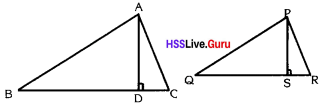ΔABC, ΔPQR are similar.
∠A = ∠P; ∠B = ∠Q;
∠C = ∠R;
∠B = ∠Q, ∠ADB = ∠PSQ = 90°
(AD ⊥ BC & PS ⊥ QR)
[each equal to 90°]
[Since the corresponding sides of similar triangles are proportional]
∴ $$\frac{AD}{PS} = \frac{DB}{SQ} = \frac{AB}{PQ} ⇒ \frac{AB}{PQ} = \frac{AD}{PS}$$

Chapter 7 Maths Class 9 Kerala Syllabus Question 6.
Prove that if the area of two similar triangles are proportional to the squares on their corresponding sides.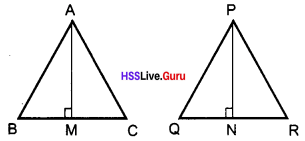ΔABC ~ ΔPQR.
AM, PN are perpendicular, $$\frac{AM}{PN} = \frac{BC}{QR}$$
Area of ΔABC = $$\frac{1}{2}$$ BC × AM
Area of ΔPQR = $$\frac{1}{2}$$ QR × PN
$$\frac{Area of ΔABC}{Area of ΔPQR}$$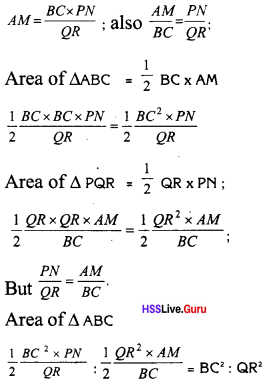Std 9 Maths Kerala Syllabus Kerala Syllabus Question 7.
In the given figure, PC ⊥ QR and QD ⊥ PR. Prove that ΔPCR and ΔQDR are similar.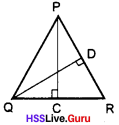In ΔPCR, ∠PCR = 90°, and in ΔQDR = 90°
∠R is common to both the triangles
ΔPCR, ΔQDR. ∠PCR = ∠QDR = 90°
∠PRC = ∠QRD (common)
(Two triangles having two pairs of corresponding angles equal. The triangles are similar.)

Kerala Syllabus 9th Standard Maths Notes Malayalam Medium Question 8.
In ΔABC, P is a point on BC. Where D, E, F are the mid-points on BP, AP and CP respectively, then prove that ΔABC ~ ΔDEF.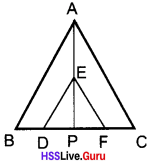Consider ΔABP, ΔDPE.
ΔABP ~ ΔDPE (∠A is ∠BAP = ∠DEP and ∠ABP = ∠EDP)
∴ $$\frac{AB}{ED} = \frac{AP}{EP}$$ …..(1)
similarly ΔAPC ≅ ΔEPF.
∴ $$\frac{AC}{EF} = \frac{AP}{EP}$$ …..(2)
(1) = (2) $$\frac{AB}{ED} = \frac{AC}{EF} = \frac{AP}{EP}$$
ΔABC ~ ΔDEF

Kerala Syllabus 9th Standard Maths Guide Pdf Question 9.
In figure AD || BC, AB || DE prove that ΔABC – ΔEDA .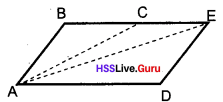∠DAE = ∠C
∠BAC = ∠DEA
(AB || DE corresponding angles)
ΔABC ~ ΔEDA

Question 10.
ΔABC is a right angled triangle. ∠B = 90° a perpendicular line from B to AC intersect AC at D. Prove that ΔABC, ΔADB and ΔBCD are similar to each other.ΔABC = ΔABD
[∠ABC = ∠ADB = 90°, ∠ACB = ∠ABD = 90 – X]
∠A (common)
similarly ΔABD ~ ΔBDC
In ΔABC, consider
∠A = X°
∠B = 90°
∠A + C = 90°, ∠C = 90 – X